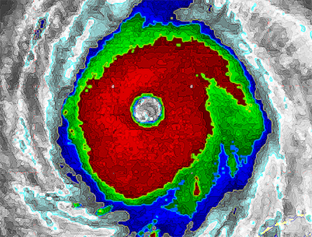## Tropical Cyclone Shear/Instability Phase Space Study

The SHIPS statistical intensity forecast model includes 21 predictors related to persistence, climatology, ocean properties and atmospheric environment variables. Most of these predictors are related in some way to either the vertical wind shear or the atmospheric instability. To better understand TC intensity change and to eventually reduce the number of inputs to the SHIPS model, the TC evolution is being studied in a simple 2-dimensional phase space. The two input variables are the wind shear of the storm environment modified by latitude (S) and the convective instability (C) as measured from an entraining vertical plume model. The vertical shear parameter S is given by S = s[1-0.8sin(lat)] where s is the 850-200 hPa vertical shear determined from NCEP GFS analyses and lat is the latitude. The constant 0.8 was determined by maximizing the correlation of observed intensity changes to S for a large sample of cases. The convective instability is the surface to 12 km average vertical velocity of a parcel of air lifted from the surface with an initial vertical velocity of 8 m/s. The parcel calculation uses an environmental sounding from NCEP GFS analyses in the storm environment, and takes into account entrainment, the weight of the condensate and the ice phase using the thermodynamic formulation of Ooyama (1990). Precipitation is crudely included by allowed the condensate to fall from the parcel at a rate with a specified e-folding time of 10 minutes.### Idealized Phase Space

The hypothesis of this study is that much of the variability of TC intensity change, and to some extent, wind structure, depends on the S-C phase space evolution. This behavior is summarized in a schematic which is available here, where the phase space is divided into quadrants based on above or below average values of S and C.

### Case Studies

Available from the navigation menu or here.

 Katrina 2005 S=low C=high rapid intensification and eye wall cycles Daniel 2006 S=low C=low annular hurricane Claudette 2003 S=high C=high transient behavior, limited intensification Debby 2000 S=very high C=high transient behavior, dissipation